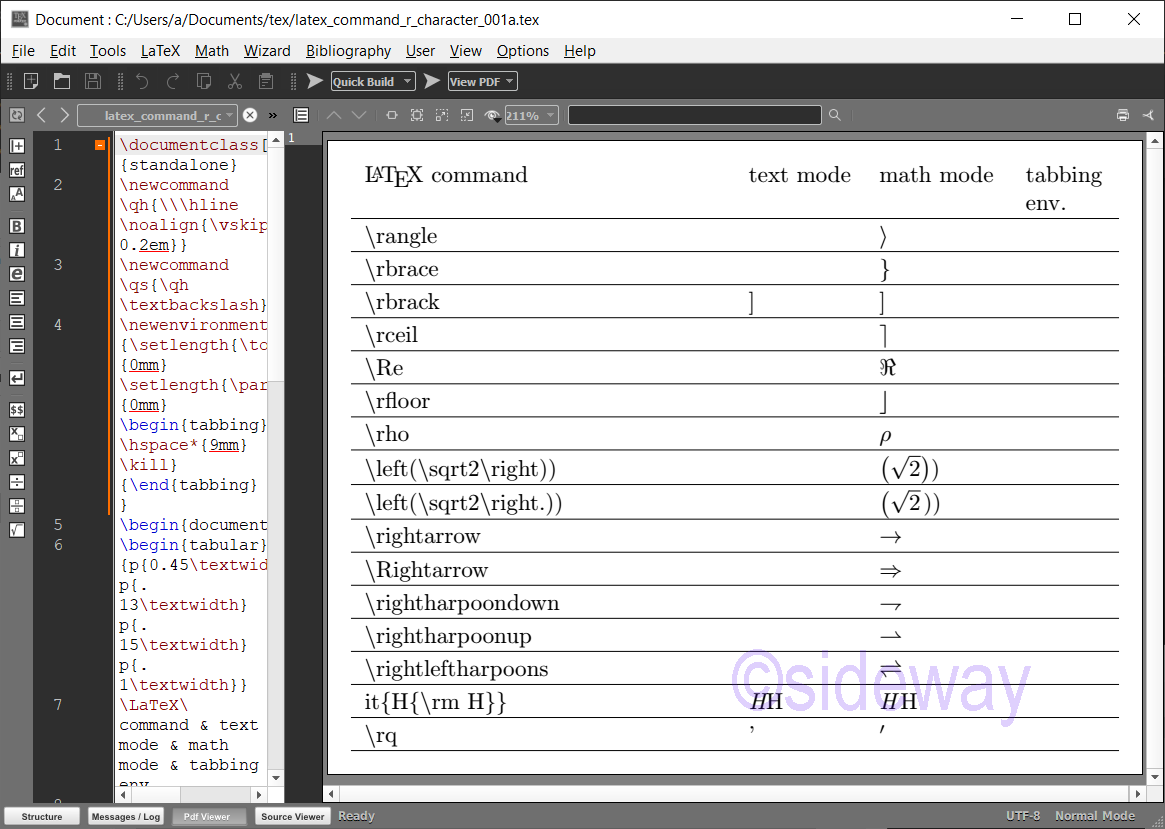output.to from Sideway
Draft for Information Only

# Content

LaTeX \r⋯ Commands for Printing Characters \r⋯ Commands \r⋯ Command Examples Source and Reference

# LaTeX \r⋯ Commands for Printing Characters

LaTeX uses backslash+r⋯ combination to print characters.

## \r⋯ Commands

The typical \r⋯ Commands for Printing Characters are LaTeX CommandsRemarksMode \rangleprints a mathematical right angle bracket, ⟩.math mode \rbraceprints a mathematical right curly bracket, }.math mode \rbrackprints a mathematical right square bracket, ].math mode \rceilprints a right ceiling, ⌉.math mode \Reprints a black-letter capital R, ℜ.math mode \rfloorprints a right floor, ⌋.math mode \rhoprints a Greek small letter rho, ρ.math mode \rightprints a dynamic delimiter depending on the content.math mode \rightprints a null delimiter for pairing with \left.math mode \rightarrowprints a rightwards arrow, →.math mode \Rightarrowprints a rightwards arrow, ⇒.math mode \rightharpoondownprints a rightwards harpoon with barb downwards, ⇁.math mode \rightharpoonupprints a rightwards harpoon with barb upwards, ⇀.math mode \rightleftharpoonsprints a rightwards harpoon over leftwards harpoon, ⇌.math mode \rmprints a Roman type characters. \rqprints a right single quotation mark, ’.math mode

## \r⋯ Command Examples

Examples of LaTeX commands
###### Code
\documentclass[border=10pt,varwidth]{standalone}
\newcommand\qh{\\\hline\noalign{\vskip 0.2em}}
\newcommand\qs{\qh\textbackslash}
\newenvironment{tabtest} {\setlength{\topsep}{0mm}\setlength{\partopsep}{0mm}\begin{tabbing}\hspace*{9mm}\kill}{\end{tabbing} }
\begin{document}
\begin{tabular}{p{0.45\textwidth}p{.13\textwidth}p{.15\textwidth}p{.1\textwidth}}
\LaTeX\ command & text mode & math mode & tabbing env.
\qs rangle&&$\rangle$
\qs rbrace&&$\rbrace$
\qs rbrack&\rbrack&$\rbrack$
\qs rceil&&$\rceil$
\qs Re&&$\Re$
\qs rfloor&&$\rfloor$
\qs rho&&$\rho$
\qs left(\textbackslash sqrt2\textbackslash right))&&$\left(\sqrt2\right))$
\qs left(\textbackslash sqrt2\textbackslash right.))&&$\left(\sqrt2\right.))$
\qs rightarrow&&$\rightarrow$
\qs Rightarrow&&$\Rightarrow$
\qs rightharpoondown&&$\rightharpoondown$
\qs rightharpoonup&&$\rightharpoonup$
\qs rightleftharpoons&&$\rightleftharpoons$
\qh it\{H\{\textbackslash rm H\}\}&\it{H{\rm H}}&$\it{H{\rm H}}$
\qs rq&\rq&$\rq$
\\\hline\end{tabular}

\end{document}
###### Output## Source and Reference

©sideway

ID: 210300003 Last Updated: 3/3/2021 Revision: 0Home 5

Business

Management

HBR 3

Information

Recreation

Culture

Chinese 1097

English 337

Computer

Hardware 153

Software

Application 204

Latex 34

Manim 171

Numeric 19

Programming

Web 285

Unicode 504

HTML 65

CSS 59

ASP.NET 194

OS 412

Python 56

Knowledge

Mathematics

Algebra 30

Geometry 21

Calculus 67

Engineering

Mechanical

Rigid Bodies

Statics 92

Dynamics 37

Control

Natural Sciences

Electric 27

Copyright © 2000-2021 Sideway . All rights reserved Disclaimers last modified on 06 September 2019